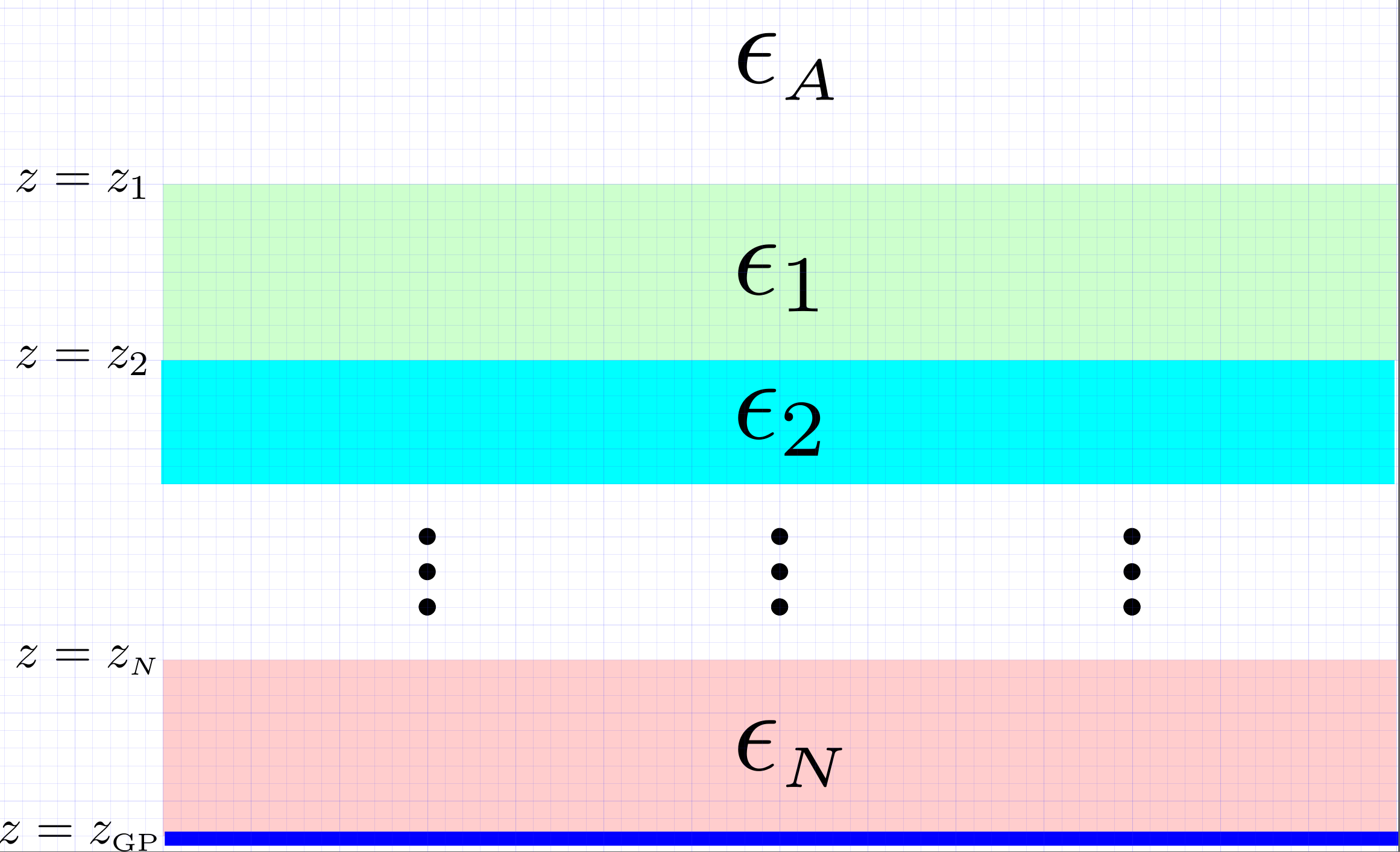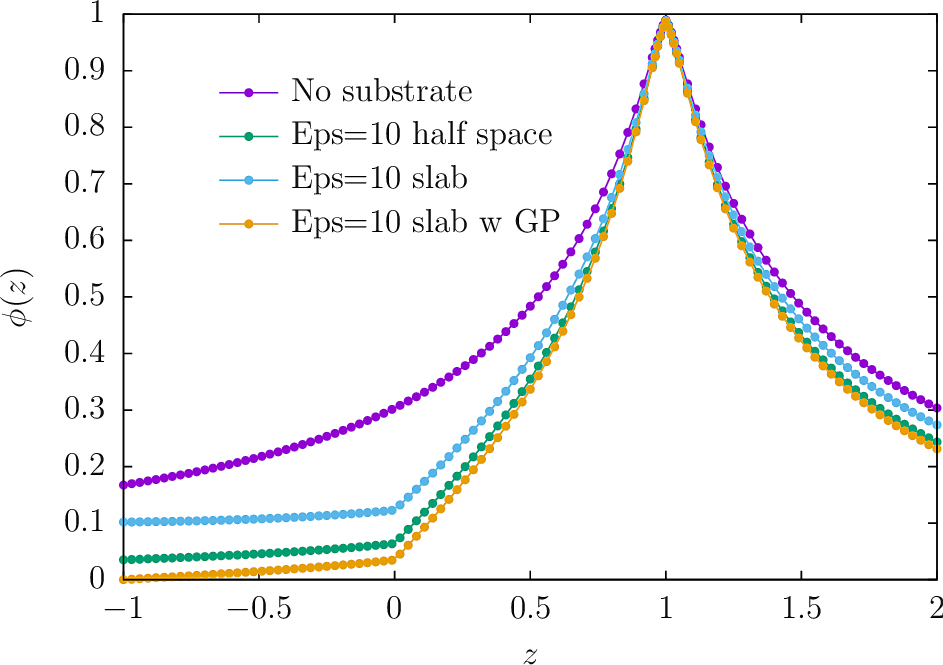Implicit treatment of layered dielectric substrates in scuff-static

In this example, we exploit scuff-static's ability to handle multilayered material substrates implicitly---with no explicit meshing of substrate layers required---to allow highly efficient modeling of the electrostatics of complex-shaped metallic traces on printed circuit boards.

The input files for the calculations discussed here may be found in the share/scuff-em/examples/ImplicitSubstrate subdirectory of your scuff-em installation.

Also, the mathematical techniques involved are discussed in this memo.

Layered substrates in scuff-em: The .substrate file

Layered substrates in scuff-em consist of zero or more planar material layers, of arbitrary user-specified thicknesses and permittivities, optionally terminated by a perfectly-conducting ground plane. All layers are infinitely extended in the x and y directions.As is clear from this figure, an N-layered substrate is fully specified by giving, for layers n=1,2,...,N,

• $z_n$, the $z$-coordinate of the upper surface of the $n$th layer
• $\epsilon_n$, the permittivity of the $n$th layer

together with

• an optional specification of the $z$-coordinate of the terminating ground plane, if present
• an optional specification of the dielectric function $\epsilon_A$ of the ambient (or "above") medium, if it is not vacuum.

This information is specified to scuff-em in the form of a simple text file conventionally given the file extension .substrate. Each line of this file [blank lines and comments (lines beginning with #) are ignored] describes one of the following items:

(a) a single layer in a multilayered substrate, specified by the z-coordinate of its upper surface and a scuff-em material designation, or

(b) the permittivity of the ambient (above) medium (if not vacuum) specified by the keyword MEDIUM followed by a scuff-em material designation, or

(c) the z-coordinate of the optional ground plane followed by the keyword GROUNDPLANE.

Examples of .substrate files

• An infinite dielectric half-space of relative permittivity $\epsilon$=10 occupying the region $z<0$:
0.0 CONST_EPS_10

• A finite-thickness (1 length unit) slab of $\epsilon=10$ suspended in space with vacuum above and below:
 0.0 CONST_EPS_10
-1.0 VACUUM

• A finite-thickness (1 length unit) slab of $\epsilon=10$ terminated below by a ground plane:
 0.0 CONST_EPS_10
-1.0 GROUNDPLANE

• An alternating stack of silicon dioxide and silicon layers above an infinitely-thick slab of silicon:
 0.0 SIO2
-0.1 SILICON
-0.2 SIO2
-0.3 SILICON


Running scuff-static calculations with implicit substrates: The --substrate option

To run electrostatics calculations in the presence of a multilayer dielectric substrate described by the file MySubstrate.substrate, simply add the option --substrate MySubstrate.substrate to the scuff-static command line.

You may set the environment variable SCUFF_SUBSTRATE_PATH to define a colon-separated list of directories in which to search for .substrate files. (The current working directory is always searched first.)

First computational example: Electric fields near a charged metallic plate

In this simple example, I consider a small finite-size metallic plate maintained at a constant potential of +1V in the vicinity of

(a) no substrate

(b) an infinite dielectric half-space of relative permittivity $\epsilon_r=10$ filling the lower half-space $z\lt 0$

(c) a finite-thickness (thickness one length unit) slab of relative permittivity $\epsilon_r=10$ filling the lower half-space $z\lt 0$

(d) a finite-thickness slab of $\epsilon_r=10$ lying atop a ground plane.

Input files for this example may be found in the share/scuff-em/examples/ImplicitSubstrate subdirectory of your scuff-em installation..

The bash script I use to run this example is RunScript:

#!/bin/bash

CODE=scuff-static

ARGS=""
ARGS="${ARGS} --geometry Square_40.scuffgeo" ARGS="${ARGS} --potentialfile  PotentialFile"
ARGS="${ARGS} --EPFile EPFile.Fields"${CODE} ${ARGS} --filebase None for SUBSTRATE in E10HalfSpace E10SlabGP E10Slab do${CODE} ${ARGS} --substrate${SUBSTRATE}.substrate --filebase \${SUBSTRATE}
done


This computes the electrostatic potential and fields along a line of evaluation points at fixed $x,y$ coordinates running from $z=2$ (above the plate) to $z=-1$ (the ground plane) and passing through the plate at $z=1$ and the dielectric interface at $z=0$. The results are plotted in gnuplot by saying load Plotter.Fields, where Plotter.Fields is this script.Take-home messages:

• The potential rises to $\phi=1$ at the location of the plate ($z=1$).

• In the presence of the $\epsilon=10$ substrate, the potential exhibits a kink at the air-substrate interface ($z=0$) that is absent for the "No substrate" curve.

• In the presence of the implicit ground plane at $z=-1$, the potential properly tends to zero at $z=-1$ (yellow curve).